# `scan` – Looping in Aesara¶

## Guide¶

The scan functions provides the basic functionality needed to do loops in Aesara. Scan comes with many whistles and bells, which we will introduce by way of examples.

### Simple loop with accumulation: Computing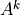¶

Assume that, given k you want to get `A**k` using a loop. More precisely, if A is a tensor you want to compute `A**k` elemwise. The python/numpy code might look like:

```result = 1
for i in range(k):
result = result * A
```

There are three things here that we need to handle: the initial value assigned to `result`, the accumulation of results in `result`, and the unchanging variable `A`. Unchanging variables are passed to scan as `non_sequences`. Initialization occurs in `outputs_info`, and the accumulation happens automatically.

The equivalent Aesara code would be:

```import aesara
import aesara.tensor as at

k = at.iscalar("k")
A = at.vector("A")

# Symbolic description of the result
result, updates = aesara.scan(fn=lambda prior_result, A: prior_result * A,
outputs_info=at.ones_like(A),
non_sequences=A,
n_steps=k)

# We only care about A**k, but scan has provided us with A**1 through A**k.
# Discard the values that we don't care about. Scan is smart enough to
# notice this and not waste memory saving them.
final_result = result[-1]

# compiled function that returns A**k

print(power(range(10),2))
print(power(range(10),4))
```
```[  0.   1.   4.   9.  16.  25.  36.  49.  64.  81.]
[  0.00000000e+00   1.00000000e+00   1.60000000e+01   8.10000000e+01
2.56000000e+02   6.25000000e+02   1.29600000e+03   2.40100000e+03
4.09600000e+03   6.56100000e+03]
```

Let us go through the example line by line. What we did is first to construct a function (using a lambda expression) that given `prior_result` and `A` returns `prior_result * A`. The order of parameters is fixed by scan: the output of the prior call to `fn` (or the initial value, initially) is the first parameter, followed by all non-sequences.

Next we initialize the output as a tensor with same shape and dtype as `A`, filled with ones. We give `A` to scan as a non sequence parameter and specify the number of steps `k` to iterate over our lambda expression.

Scan returns a tuple containing our result (`result`) and a dictionary of updates (empty in this case). Note that the result is not a matrix, but a 3D tensor containing the value of `A**k` for each step. We want the last value (after `k` steps) so we compile a function to return just that. Note that there is an optimization, that at compile time will detect that you are using just the last value of the result and ensure that scan does not store all the intermediate values that are used. So do not worry if `A` and `k` are large.

### Iterating over the first dimension of a tensor: Calculating a polynomial¶

In addition to looping a fixed number of times, scan can iterate over the leading dimension of tensors (similar to Python’s `for x in a_list`).

The tensor(s) to be looped over should be provided to scan using the `sequence` keyword argument.

Here’s an example that builds a symbolic calculation of a polynomial from a list of its coefficients:

```import numpy

coefficients = aesara.tensor.vector("coefficients")
x = at.scalar("x")

max_coefficients_supported = 10000

# Generate the components of the polynomial
components, updates = aesara.scan(fn=lambda coefficient, power, free_variable: coefficient * (free_variable ** power),
outputs_info=None,
sequences=[coefficients, aesara.tensor.arange(max_coefficients_supported)],
non_sequences=x)
# Sum them up
polynomial = components.sum()

# Compile a function
calculate_polynomial = aesara.function(inputs=[coefficients, x], outputs=polynomial)

# Test
test_coefficients = numpy.asarray([1, 0, 2], dtype=numpy.float32)
test_value = 3
print(calculate_polynomial(test_coefficients, test_value))
print(1.0 * (3 ** 0) + 0.0 * (3 ** 1) + 2.0 * (3 ** 2))
```
```19.0
19.0
```

There are a few things to note here.

First, we calculate the polynomial by first generating each of the coefficients, and then summing them at the end. (We could also have accumulated them along the way, and then taken the last one, which would have been more memory-efficient, but this is an example.)

Second, there is no accumulation of results, we can set `outputs_info` to `None`. This indicates to scan that it doesn’t need to pass the prior result to `fn`.

The general order of function parameters to `fn` is:

```sequences (if any), prior result(s) (if needed), non-sequences (if any)
```

Third, there’s a handy trick used to simulate python’s `enumerate`: simply include `aesara.tensor.arange` to the sequences.

Fourth, given multiple sequences of uneven lengths, scan will truncate to the shortest of them. This makes it safe to pass a very long arange, which we need to do for generality, since arange must have its length specified at creation time.

### Simple accumulation into a scalar, ditching lambda¶

Although this example would seem almost self-explanatory, it stresses a pitfall to be careful of: the initial output state that is supplied, that is `outputs_info`, must be of a shape similar to that of the output variable generated at each iteration and moreover, it must not involve an implicit downcast of the latter.

```import numpy as np
import aesara
import aesara.tensor as at

up_to = at.iscalar("up_to")

# define a named function, rather than using lambda
return sum_to_date + arange_val
seq = at.arange(up_to)

# An unauthorized implicit downcast from the dtype of 'seq', to that of
# 'at.as_tensor_variable(0)' which is of dtype 'int8' by default would occur
# if this instruction were to be used instead of the next one:
# outputs_info = at.as_tensor_variable(0)

outputs_info = at.as_tensor_variable(np.asarray(0, seq.dtype))
outputs_info=outputs_info,
sequences=seq)
triangular_sequence = aesara.function(inputs=[up_to], outputs=scan_result)

# test
some_num = 15
print(triangular_sequence(some_num))
print([n * (n + 1) // 2 for n in range(some_num)])
```
```[  0   1   3   6  10  15  21  28  36  45  55  66  78  91 105]
[0, 1, 3, 6, 10, 15, 21, 28, 36, 45, 55, 66, 78, 91, 105]
```

### Another simple example¶

Unlike some of the prior examples, this one is hard to reproduce except by using scan.

This takes a sequence of array indices, and values to place there, and a “model” output array (whose shape and dtype will be mimicked), and produces a sequence of arrays with the shape and dtype of the model, with all values set to zero except at the provided array indices.

```location = at.imatrix("location")
values = at.vector("values")
output_model = at.matrix("output_model")

def set_value_at_position(a_location, a_value, output_model):
zeros = at.zeros_like(output_model)
zeros_subtensor = zeros[a_location, a_location]
return at.set_subtensor(zeros_subtensor, a_value)

outputs_info=None,
sequences=[location, values],
non_sequences=output_model)

assign_values_at_positions = aesara.function(inputs=[location, values, output_model], outputs=result)

# test
test_locations = numpy.asarray([[1, 1], [2, 3]], dtype=numpy.int32)
test_values = numpy.asarray([42, 50], dtype=numpy.float32)
test_output_model = numpy.zeros((5, 5), dtype=numpy.float32)
print(assign_values_at_positions(test_locations, test_values, test_output_model))
```
```[[[  0.   0.   0.   0.   0.]
[  0.  42.   0.   0.   0.]
[  0.   0.   0.   0.   0.]
[  0.   0.   0.   0.   0.]
[  0.   0.   0.   0.   0.]]

[[  0.   0.   0.   0.   0.]
[  0.   0.   0.   0.   0.]
[  0.   0.   0.  50.   0.]
[  0.   0.   0.   0.   0.]
[  0.   0.   0.   0.   0.]]]
```

This demonstrates that you can introduce new Aesara variables into a scan function.

### Using shared variables - Gibbs sampling¶

Another useful feature of scan, is that it can handle shared variables. For example, if we want to implement a Gibbs chain of length 10 we would do the following:

```import aesara
from aesara import tensor as at

W = aesara.shared(W_values) # we assume that ``W_values`` contains the
# initial values of your weight matrix

bvis = aesara.shared(bvis_values)
bhid = aesara.shared(bhid_values)

trng = aesara.tensor.random.utils.RandomStream(1234)

def OneStep(vsample) :
hmean = at.sigmoid(aesara.dot(vsample, W) + bhid)
hsample = trng.binomial(size=hmean.shape, n=1, p=hmean)
vmean = at.sigmoid(aesara.dot(hsample, W.T) + bvis)
return trng.binomial(size=vsample.shape, n=1, p=vmean,
dtype=aesara.config.floatX)

sample = aesara.tensor.vector()

values, updates = aesara.scan(OneStep, outputs_info=sample, n_steps=10)

```

The first, and probably most crucial observation is that the updates dictionary becomes important in this case. It links a shared variable with its updated value after k steps. In this case it tells how the random streams get updated after 10 iterations. If you do not pass this update dictionary to your function, you will always get the same 10 sets of random numbers. You can even use the `updates` dictionary afterwards. Look at this example :

```a = aesara.shared(1)
values, updates = aesara.scan(lambda: {a: a+1}, n_steps=10)
```

In this case the lambda expression does not require any input parameters and returns an update dictionary which tells how `a` should be updated after each step of scan. If we write :

```b = a + 1

print(b)
print(c)
print(a.get_value())
```

We will see that because `b` does not use the updated version of `a`, it will be 2, `c` will be 12, while `a.value` is `11`. If we call the function again, `b` will become 12, `c` will be 22 and `a.value` 21. If we do not pass the `updates` dictionary to the function, then `a.value` will always remain 1, `b` will always be 2 and `c` will always be `12`.

The second observation is that if we use shared variables ( `W`, `bvis`, `bhid`) but we do not iterate over them (i.e. scan doesn’t really need to know anything in particular about them, just that they are used inside the function applied at each step) you do not need to pass them as arguments. Scan will find them on its own and add them to the graph. However, passing them to the scan function is a good practice, as it avoids Scan Op calling any earlier (external) Op over and over. This results in a simpler computational graph, which speeds up the optimization and the execution. To pass the shared variables to Scan you need to put them in a list and give it to the `non_sequences` argument. Here is the Gibbs sampling code updated:

```W = aesara.shared(W_values) # we assume that ``W_values`` contains the
# initial values of your weight matrix

bvis = aesara.shared(bvis_values)
bhid = aesara.shared(bhid_values)

trng = aesara.tensor.random.utils.RandomStream(1234)

# OneStep, with explicit use of the shared variables (W, bvis, bhid)
def OneStep(vsample, W, bvis, bhid):
hmean = at.sigmoid(aesara.dot(vsample, W) + bhid)
hsample = trng.binomial(size=hmean.shape, n=1, p=hmean)
vmean = at.sigmoid(aesara.dot(hsample, W.T) + bvis)
return trng.binomial(size=vsample.shape, n=1, p=vmean,
dtype=aesara.config.floatX)

sample = aesara.tensor.vector()

# The new scan, with the shared variables passed as non_sequences
outputs_info=sample,
non_sequences=[W, bvis, bhid],
n_steps=10)

```

### Using shared variables - the strict flag¶

As we just saw, passing the shared variables to scan may result in a simpler computational graph, which speeds up the optimization and the execution. A good way to remember to pass every shared variable used during scan is to use the `strict` flag. When set to true, scan checks that all the necessary shared variables in `fn` are passed as explicit arguments to `fn`. This has to be ensured by the user. Otherwise, it will result in an error.

Using the original Gibbs sampling example, with `strict=True` added to the `scan()` call:

```# Same OneStep as in original example.
def OneStep(vsample) :
hmean = at.sigmoid(aesara.dot(vsample, W) + bhid)
hsample = trng.binomial(size=hmean.shape, n=1, p=hmean)
vmean = at.sigmoid(aesara.dot(hsample, W.T) + bvis)
return trng.binomial(size=vsample.shape, n=1, p=vmean,
dtype=aesara.config.floatX)

# The new scan, adding strict=True to the original call.
outputs_info=sample,
n_steps=10,
strict=True)
```
```Traceback (most recent call last):
...
MissingInputError: An input of the graph, used to compute
DimShuffle{1,0}(<TensorType(float64, (None, None))>), was not provided and
not given a value.Use the Aesara flag exception_verbosity='high',for
```

The error indicates that `OneStep` relies on variables that are not passed as arguments explicitly. Here is the correct version, with the shared variables passed explicitly to `OneStep` and to scan:

```# OneStep, with explicit use of the shared variables (W, bvis, bhid)
def OneStep(vsample, W, bvis, bhid) :
hmean = at.sigmoid(aesara.dot(vsample, W) + bhid)
hsample = trng.binomial(size=hmean.shape, n=1, p=hmean)
vmean = at.sigmoid(aesara.dot(hsample, W.T) + bvis)
return trng.binomial(size=vsample.shape, n=1, p=vmean,
dtype=aesara.config.floatX)

# The new scan, adding strict=True to the original call, and passing
# explicitly W, bvis and bhid.
outputs_info=sample,
non_sequences=[W, bvis, bhid],
n_steps=10,
strict=True)
```

### Multiple outputs, several taps values - Recurrent Neural Network with Scan¶

The examples above showed simple uses of scan. However, scan also supports referring not only to the prior result and the current sequence value, but also looking back more than one step.

This is needed, for example, to implement a RNN using scan. Assume that our RNN is defined as follows :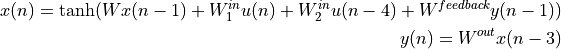Note that this network is far from a classical recurrent neural network and might be useless. The reason we defined as such is to better illustrate the features of scan.

In this case we have a sequence over which we need to iterate `u`, and two outputs `x` and `y`. To implement this with scan we first construct a function that computes one iteration step :

```def oneStep(u_tm4, u_t, x_tm3, x_tm1, y_tm1, W, W_in_1, W_in_2,  W_feedback, W_out):

x_t = at.tanh(aesara.dot(x_tm1, W) + \
aesara.dot(u_t,   W_in_1) + \
aesara.dot(u_tm4, W_in_2) + \
aesara.dot(y_tm1, W_feedback))
y_t = aesara.dot(x_tm3, W_out)

return [x_t, y_t]
```

As naming convention for the variables we used `a_tmb` to mean `a` at `t-b` and `a_tpb` to be `a` at `t+b`. Note the order in which the parameters are given, and in which the result is returned. Try to respect chronological order among the taps ( time slices of sequences or outputs) used. For scan is crucial only for the variables representing the different time taps to be in the same order as the one in which these taps are given. Also, not only taps should respect an order, but also variables, since this is how scan figures out what should be represented by what. Given that we have all the Aesara variables needed we construct our RNN as follows :

```W = at.matrix()
W_in_1 = at.matrix()
W_in_2 = at.matrix()
W_feedback = at.matrix()
W_out = at.matrix()

u = at.matrix() # it is a sequence of vectors
x0 = at.matrix() # initial state of x has to be a matrix, since
# it has to cover x[-3]
y0 = at.vector() # y0 is just a vector since scan has only to provide
# y[-1]

sequences=dict(input=u, taps=[-4,-0]),
outputs_info=[dict(initial=x0, taps=[-3,-1]), y0],
non_sequences=[W, W_in_1, W_in_2, W_feedback, W_out],
strict=True)
# for second input y, scan adds -1 in output_taps by default
```

Now `x_vals` and `y_vals` are symbolic variables pointing to the sequence of x and y values generated by iterating over u. The `sequence_taps`, `outputs_taps` give to scan information about what slices are exactly needed. Note that if we want to use `x[t-k]` we do not need to also have `x[t-(k-1)], x[t-(k-2)],..`, but when applying the compiled function, the numpy array given to represent this sequence should be large enough to cover this values. Assume that we compile the above function, and we give as `u` the array `uvals = [0,1,2,3,4,5,6,7,8]`. By abusing notations, scan will consider `uvals` as `u[-4]`, and will start scanning from `uvals` towards the end.

### Conditional ending of Scan¶

Scan can also be used as a `repeat-until` block. In such a case scan will stop when either the maximal number of iteration is reached, or the provided condition evaluates to True.

For an example, we will compute all powers of two smaller then some provided value `max_value`.

```def power_of_2(previous_power, max_value):
return previous_power*2, aesara.scan.utils.until(previous_power*2 > max_value)

max_value = at.scalar()
values, _ = aesara.scan(power_of_2,
outputs_info = at.constant(1.),
non_sequences = max_value,
n_steps = 1024)

f = aesara.function([max_value], values)

print(f(45))
```
```[  2.   4.   8.  16.  32.  64.]
```

As you can see, in order to terminate on condition, the only thing required is that the inner function `power_of_2` to return also the condition wrapped in the class `aesara.scan.utils.until`. The condition has to be expressed in terms of the arguments of the inner function (in this case `previous_power` and `max_value`).

As a rule, scan always expects the condition to be the last thing returned by the inner function, otherwise an error will be raised.

### Reducing Scan’s memory usage¶

This section presents the `scan_checkpoints` function. In short, this function reduces the memory usage of scan (at the cost of more computation time) by not keeping in memory all the intermediate time steps of the loop, and recomputing them when computing the gradients. This function is therefore only useful if you need to compute the gradient of the output of scan with respect to its inputs, and shouldn’t be used otherwise.

Before going more into the details, here are its current limitations:

• It only works in the case where only the output of the last time step is needed, like when computing `A**k` or in an `encoder-decoder` setup.
• It only accepts sequences of the same length.
• If `n_steps` is specified, it has the same value as the length of any sequences.
• It is singly-recurrent, meaning that only the previous time step can be used to compute the current one (i.e. `h[t]` can only depend on `h[t-1]`). In other words, `taps` can not be used in `sequences` and `outputs_info`.

Often, in order to be able to compute the gradients through scan operations, Aesara needs to keep in memory some intermediate computations of scan. This can sometimes use a prohibitively large amount of memory. `scan_checkpoints` allows to discard some of those intermediate steps and recompute them again when computing the gradients. Its `save_every_N` argument specifies the number time steps to do without storing the intermediate results. For example, `save_every_N = 4` will reduce the memory usage by 4, while having to recompute 3/4 time steps of the forward loop. Since the grad of scan is about 6x slower than the forward, a ~20% slowdown is expected. Apart from the `save_every_N` argument and the current limitations, the usage of this function is similar to the classic `scan` function.

### Optimizing Scan’s performance¶

This section covers some ways to improve performance of an Aesara function using Scan.

#### Minimizing Scan usage¶

Scan makes it possible to define simple and compact graphs that can do the same work as much larger and more complicated graphs. However, it comes with a significant overhead. As such, when performance is the objective, a good rule of thumb is to perform as much of the computation as possible outside of Scan. This may have the effect of increasing memory usage but can also reduce the overhead introduces by using Scan.

#### Explicitly passing inputs of the inner function to scan¶

It is possible, inside of Scan, to use variables previously defined outside of the Scan without explicitly passing them as inputs to the Scan. However, it is often more efficient to explicitly pass them as non-sequence inputs instead. Section Using shared variables - Gibbs sampling provides an explanation for this and section Using shared variables - the strict flag describes the strict flag, a tool that Scan provides to help ensure that the inputs to the function inside Scan have all been provided as explicit inputs to the `scan()` function.

#### Deactivating garbage collecting in Scan¶

Deactivating the garbage collection for Scan can allow it to reuse memory between executions instead of always having to allocate new memory. This can improve performance at the cost of increased memory usage. By default, Scan reuses memory between iterations of the same execution but frees the memory after the last iteration.

There are two ways to achieve this, using the Aesara flag `config.scan__allow_gc` and setting it to False, or using the argument `allow_gc` of the function aesara.scan() and set it to False (when a value is not provided for this argument, the value of the flag `config.scan__allow_gc` is used).

#### Graph optimizations¶

This one is simple but still worth pointing out. Aesara is able to automatically recognize and optimize many computation patterns. However, there are patterns that Aesara doesn’t optimize because doing so would change the user interface (such as merging shared variables together into a single one, for instance). Additionally, Aesara doesn’t catch every case that it could optimize and so it remains useful for performance that the user defines an efficient graph in the first place. This is also the case, and sometimes even more so, for the graph inside of Scan. This is because it will be executed many times for every execution of the Aesara function that contains it.

The LSTM tutorial on DeepLearning.net provides an example of an optimization that Aesara cannot perform. Instead of performing many matrix multiplications between matrix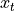and each of the shared matrices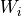,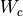,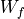and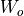, the matrices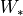, are merged into a single shared matrix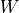and the graph performs a single larger matrix multiplication betweenand. The resulting matrix is then sliced to obtain the results of that the small individual matrix multiplications would have produced. This optimization replaces several small and inefficient matrix multiplications by a single larger one and thus improves performance at the cost of a potentially higher memory usage.

## reference¶

This module provides the Scan Op.

Scanning is a general form of recurrence, which can be used for looping. The idea is that you scan a function along some input sequence, producing an output at each time-step that can be seen (but not modified) by the function at the next time-step. (Technically, the function can see the previous K time-steps of your outputs and L time steps (from past and future) of your inputs.

So for example, `sum()` could be computed by scanning the `z+x_i` function over a list, given an initial state of `z=0`.

Special cases:

• A reduce operation can be performed by using only the last output of a `scan`.
• A map operation can be performed by applying a function that ignores previous steps of the outputs.

Often a for-loop or while-loop can be expressed as a `scan()` operation, and `scan` is the closest that aesara comes to looping. The advantages of using `scan` over `for` loops in python (among others) are:

• it allows the number of iterations to be part of the symbolic graph
• it allows computing gradients through the for loop
• there exist a bunch of optimizations that help re-write your loop such that less memory is used and that it runs faster

The Scan Op should typically be used by calling any of the following functions: `scan()`, `map()`, `reduce()`, `foldl()`, `foldr()`.

`aesara.``map`(fn, sequences, non_sequences=None, truncate_gradient=- 1, go_backwards=False, mode=None, name=None)[source]

Construct a `Scan` `Op` that functions like `map`.

Parameters: fn – The function that `map` applies at each iteration step (see `scan` for more info). sequences – List of sequences over which `map` iterates (see `scan` for more info). non_sequences – List of arguments passed to `fn`. `map` will not iterate over these arguments (see `scan` for more info). truncate_gradient – See `scan`. go_backwards (bool) – Decides the direction of iteration. True means that sequences are parsed from the end towards the beginning, while False is the other way around. mode – See `scan`. name – See `scan`.
`aesara.``reduce`(fn, sequences, outputs_info, non_sequences=None, go_backwards=False, mode=None, name=None)[source]

Construct a `Scan` `Op` that functions like `reduce`.

Parameters: fn – The function that `reduce` applies at each iteration step (see `scan` for more info). sequences – List of sequences over which `reduce` iterates (see `scan` for more info). outputs_info – List of dictionaries describing the outputs of reduce (see `scan` for more info). non_sequences – List of arguments passed to `fn`. `reduce` willnot iterate over these arguments (see `scan` for more info). go_backwards (bool) – Decides the direction of iteration. True means that sequences are parsed from the end towards the beginning, while False is the other way around. mode – See `scan`. name – See `scan`.
`aesara.``foldl`(fn, sequences, outputs_info, non_sequences=None, mode=None, name=None)[source]

Construct a `Scan` `Op` that functions like Haskell’s `foldl`.

Parameters: fn – The function that `foldl` applies at each iteration step (see `scan` for more info). sequences – List of sequences over which `foldl` iterates (see `scan` for more info). outputs_info – List of dictionaries describing the outputs of reduce (see `scan` for more info). non_sequences – List of arguments passed to `fn`. `foldl` will not iterate over these arguments (see `scan` for more info). mode – See `scan`. name – See `scan`.
`aesara.``foldr`(fn, sequences, outputs_info, non_sequences=None, mode=None, name=None)[source]

Construct a `Scan` `Op` that functions like Haskell’s `foldr`.

Parameters: fn – The function that `foldr` applies at each iteration step (see `scan` for more info). sequences – List of sequences over which `foldr` iterates (see `scan` for more info). outputs_info – List of dictionaries describing the outputs of reduce (see `scan` for more info). non_sequences – List of arguments passed to `fn`. `foldr` will not iterate over these arguments (see `scan` for more info). mode – See `scan`. name – See `scan`.
`aesara.``scan`(fn, sequences=None, outputs_info=None, non_sequences=None, n_steps=None, truncate_gradient=- 1, go_backwards=False, mode=None, name=None, profile=False, allow_gc=None, strict=False, return_list=False)[source]

This function constructs and applies a `Scan` `Op` to the provided arguments.

Parameters: fn – `fn` is a function that describes the operations involved in one step of `scan`. `fn` should construct variables describing the output of one iteration step. It should expect as input `Variable`s representing all the slices of the input sequences and previous values of the outputs, as well as all other arguments given to scan as `non_sequences`. The order in which scan passes these variables to `fn` is the following : all time slices of the first sequence all time slices of the second sequence … all time slices of the last sequence all past slices of the first output all past slices of the second output … all past slices of the last output all other arguments (the list given as `non_sequences` to`scan`) The order of the sequences is the same as the one in the list `sequences` given to `scan`. The order of the outputs is the same as the order of `outputs_info`. For any sequence or output the order of the time slices is the same as the one in which they have been given as taps. For example if one writes the following : ```scan(fn, sequences = [ dict(input= Sequence1, taps = [-3,2,-1]) , Sequence2 , dict(input = Sequence3, taps = 3) ] , outputs_info = [ dict(initial = Output1, taps = [-3,-5]) , dict(initial = Output2, taps = None) , Output3 ] , non_sequences = [ Argument1, Argument2]) ``` `fn` should expect the following arguments in this given order: `sequence1[t-3]` `sequence1[t+2]` `sequence1[t-1]` `sequence2[t]` `sequence3[t+3]` `output1[t-3]` `output1[t-5]` `output3[t-1]` `argument1` `argument2` The list of `non_sequences` can also contain shared variables used in the function, though `scan` is able to figure those out on its own so they can be skipped. For the clarity of the code we recommend though to provide them to `scan`. To some extend `scan` can also figure out other `non sequences` (not shared) even if not passed to `scan` (but used by `fn`). A simple example of this would be : ```import aesara.tensor as at W = at.matrix() W_2 = W**2 def f(x): return at.dot(x,W_2) ``` The function `fn` is expected to return two things. One is a list of outputs ordered in the same order as `outputs_info`, with the difference that there should be only one output variable per output initial state (even if no tap value is used). Secondly `fn` should return an update dictionary (that tells how to update any shared variable after each iteration step). The dictionary can optionally be given as a list of tuples. There is no constraint on the order of these two list, `fn` can return either `(outputs_list, update_dictionary)` or `(update_dictionary, outputs_list)` or just one of the two (in case the other is empty). To use `scan` as a `while` loop, the user needs to change the function `fn` such that also a stopping condition is returned. To do so, one needs to wrap the condition in an `until` class. The condition should be returned as a third element, for example: ```... return [y1_t, y2_t], {x:x+1}, until(x < 50) ``` Note that a number of steps–considered in here as the maximum number of steps–is still required even though a condition is passed. It is used to allocate memory if needed. sequences – `sequences` is the list of `Variable`s or `dict`s describing the sequences `scan` has to iterate over. If a sequence is given as wrapped in a `dict`, then a set of optional information can be provided about the sequence. The `dict` should have the following keys: `input` (mandatory) – `Variable` representing the sequence. `taps` – Temporal taps of the sequence required by `fn`. They are provided as a list of integers, where a value `k` impiles that at iteration step `t` scan will pass to `fn` the slice `t+k`. Default value is `` All `Variable`s in the list `sequences` are automatically wrapped into a `dict` where `taps` is set to `` outputs_info – `outputs_info` is the list of `Variable`s or `dict`s describing the initial state of the outputs computed recurrently. When the initial states are given as `dict`s, optional information can be provided about the output corresponding to those initial states. The `dict` should have the following keys: `initial` – A `Variable` that represents the initial state of a given output. In case the output is not computed recursively (e.g. a `map`-like function) and does not require an initial state, this field can be skipped. Given that only the previous time step of the output is used by `fn`, the initial state should have the same shape as the output and should not involve a downcast of the data type of the output. If multiple time taps are used, the initial state should have one extra dimension that covers all the possible taps. For example if we use `-5`, `-2` and `-1` as past taps, at step `0`, `fn` will require (by an abuse of notation) `output[-5]`, `output[-2]` and `output[-1]`. This will be given by the initial state, which in this case should have the shape `(5,) + output.shape`. If this `Variable` containing the initial state is called `init_y` then `init_y` corresponds to `output[-5]`. `init_y` corresponds to `output[-4]`, `init_y` corresponds to `output[-3]`, `init_y` corresponds to `output[-2]`, `init_y` corresponds to `output[-1]`. While this order might seem strange, it comes natural from splitting an array at a given point. assume that we have a array `x`, and we choose `k` to be time step `0`. Then our initial state would be `x[:k]`, while the output will be `x[k:]`. Looking at this split, elements in `x[:k]` are ordered exactly like those in `init_y`. `taps` – Temporal taps of the output that will be passed to `fn`. They are provided as a list of negative integers, where a value `k` implies that at iteration step `t` scan will pass to `fn` the slice `t+k`. `scan` will follow this logic if partial information is given: If an output is not wrapped in a `dict`, `scan` will wrap it in one assuming that you use only the last step of the output (i.e. it makes your tap value list equal to `[-1]`). If you wrap an output in a `dict` and you do not provide any taps but you provide an initial state it will assume that you are using only a tap value of `-1`. If you wrap an output in a `dict` but you do not provide any initial state, it assumes that you are not using any form of taps. If you provide a `None` instead of a `Variable` or a empty `dict` `scan` assumes that you will not use any taps for this output (like for example in case of a `map`) If `outputs_info` is an empty `list` or `None`, `scan` assumes that no tap is used for any of the outputs. If information is provided just for a subset of the outputs, an exception is raised, because there is no convention on how scan should map the provided information to the outputs of `fn`. non_sequences – `non_sequences` is the list of arguments that are passed to `fn` at each steps. One can choose to exclude variables used in `fn` from this list, as long as they are part of the computational graph, although–for clarity–this is not encouraged. n_steps – `n_steps` is the number of steps to iterate given as an `int` or a scalar `Variable`. If any of the input sequences do not have enough elements, `scan` will raise an error. If the value is `0`, the outputs will have `0` rows. If `n_steps` is not provided, `scan` will figure out the amount of steps it should run given its input sequences. `n_steps < 0` is not supported anymore. truncate_gradient – `truncate_gradient` is the number of steps to use in truncated back-propagation through time (BPTT). If you compute gradients through a `Scan` `Op`, they are computed using BPTT. By providing a different value then `-1`, you choose to use truncated BPTT instead of classical BPTT, where you go for only `truncate_gradient` number of steps back in time. go_backwards – `go_backwards` is a flag indicating if `scan` should go backwards through the sequences. If you think of each sequence as indexed by time, making this flag `True` would mean that `scan` goes back in time, namely that for any sequence it starts from the end and goes towards `0`. name – When profiling `scan`, it is helpful to provide a name for any instance of `scan`. For example, the profiler will produce an overall profile of your code as well as profiles for the computation of one step of each instance of `Scan`. The `name` of the instance appears in those profiles and can greatly help to disambiguate information. mode – The mode used to compile the inner-graph. If you prefer the computations of one step of `scan` to be done differently then the entire function, you can use this parameter to describe how the computations in this loop are done (see `aesara.function` for details about possible values and their meaning). profile – If `True` or a non-empty string, a profile object will be created and attached to the inner graph of `Scan`. When `profile` is `True`, the profiler results will use the name of the `Scan` instance, otherwise it will use the passed string. The profiler only collects and prints information when running the inner graph with the `CVM` `Linker`. allow_gc – Set the value of `allow_gc` for the internal graph of the `Scan`. If set to `None`, this will use the value of `aesara.config.scan__allow_gc`. The full `Scan` behavior related to allocation is determined by this value and the flag `aesara.config.allow_gc`. If the flag `allow_gc` is `True` (default) and this `allow_gc` is `False` (default), then we let `Scan` allocate all intermediate memory on the first iteration, and they are not garbage collected after that first iteration; this is determined by `allow_gc`. This can speed up allocation of the subsequent iterations. All those temporary allocations are freed at the end of all iterations; this is what the flag `aesara.config.allow_gc` means. strict – If `True`, all the shared variables used in `fn` must be provided as a part of `non_sequences` or `sequences`. return_list – If `True`, will always return a `list`, even if there is only one output. `tuple` of the form `(outputs, updates)`. `outputs` is either a `Variable` or a `list` of `Variable`s representing the outputs in the same order as in `outputs_info`. `updates` is a subclass of `dict` specifying the update rules for all shared variables used in `Scan`. This `dict` should be passed to `aesara.function` when you compile your function. tuple
`aesara.scan.``scan_checkpoints`(fn, sequences=None, outputs_info=None, non_sequences=None, name='checkpointscan_fn', n_steps=None, save_every_N=10, padding=True)[source]

Scan function that uses less memory, but is more restrictive.

In `scan()`, if you compute the gradient of the output with respect to the input, you will have to store the intermediate results at each time step, which can be prohibitively huge. This function allows to do `save_every_N` steps of forward computations without storing the intermediate results, and to recompute them during the gradient computation.

Notes

Current assumptions:

• Every sequence has the same length.
• If `n_steps` is specified, it has the same value as the length of any sequence.
• The value of `save_every_N` divides the number of steps the scan will run without remainder.
• Only singly-recurrent and non-recurrent outputs are used. No multiple recurrences.
• Only the last timestep of any output will ever be used.
Parameters: fn – `fn` is a function that describes the operations involved in one step of `scan`. See the documentation of `scan()` for more information. sequences – `sequences` is the list of Aesara variables or dictionaries describing the sequences `scan` has to iterate over. All sequences must be the same length in this version of `scan`. outputs_info – `outputs_info` is the list of Aesara variables or dictionaries describing the initial state of the outputs computed recurrently. non_sequences – `non_sequences` is the list of arguments that are passed to `fn` at each steps. One can opt to exclude variable used in `fn` from this list as long as they are part of the computational graph, though for clarity we encourage not to do so. n_steps – `n_steps` is the number of steps to iterate given as an int or Aesara scalar (> 0). If any of the input sequences do not have enough elements, scan will raise an error. If n_steps is not provided, `scan` will figure out the amount of steps it should run given its input sequences. save_every_N – `save_every_N` is the number of steps to go without storing the computations of `scan` (ie they will have to be recomputed during the gradient computation). padding – If the length of the sequences is not a multiple of `save_every_N`, the sequences will be zero padded to make this version of `scan` work properly, but will also result in a memory copy. It can be avoided by setting `padding` to False, but you need to make sure the length of the sequences is a multiple of `save_every_N`. Tuple of the form `(outputs, updates)` as in `scan()`, but with a small change: It only contain the output at each `save_every_N` step. The time steps that are not returned by this function will be recomputed during the gradient computation (if any). tuple

`scan()`